# Density of a probability distribution

probability density

The derivative of the distribution function corresponding to an absolutely-continuous probability measure.

Letbe a random vector taking values in an-dimensional Euclidean space, letbe its distribution function, and let there exist a non-negative functionsuch that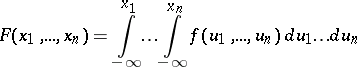for any real. Thenis called the probability density of, and for any Borel set,Any non-negative integrable functionsatisfy the condition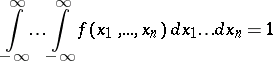is the probability density of some random vector.

If two random vectorsandtaking values inare independent and have probability densitiesandrespectively, then the random vector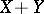has the probability densitythat is the convolution ofand: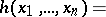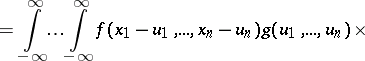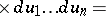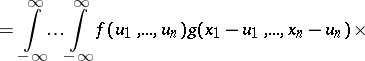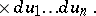Let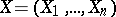and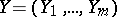be random vectors taking values inand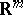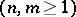and having probability densitiesandrespectively, and letbe a random vector in. If thenandare independent,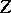has the probability density, which is called the joint probability density of the random vectorsand, where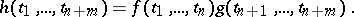(1)

Conversely, ifhas a probability density that satisfies (1), thenandare independent.

The characteristic functionof a random vectorhaving a probability densityis expressed by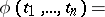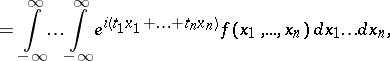where ifis absolutely integrable thenis a bounded continuous function, and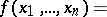The probability densityand the corresponding characteristic functionare related also by the following relation (Plancherel's identity): The function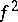is integrable if and only if the function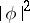is integrable, and in that case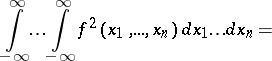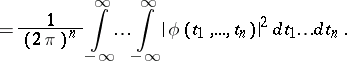Letbe a measurable space, and letandbe-finite measures on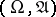withabsolutely continuous with respect to, i.e.implies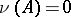,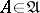. In that case there exists ona non-negative measurable functionsuch that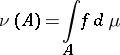for any. The functionis called the Radon–Nikodým derivative ofwith respect to, while ifis a probability measure, it is also the probability density ofrelative to.

A concept closely related to the probability density is that of a dominated family of distributions. A family of probability distributions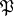on a measurable spaceis called dominated if there exists a-finite measureonsuch that each probability measure fromhas a probability density relative to(or, what is the same, if each measure fromis absolutely continuous with respect to). The assumption of dominance is important in certain theorems in mathematical statistics.

How to Cite This Entry:
Density of a probability distribution. Encyclopedia of Mathematics. URL: http://encyclopediaofmath.org/index.php?title=Density_of_a_probability_distribution&oldid=46629
This article was adapted from an original article by N.G. Ushakov (originator), which appeared in Encyclopedia of Mathematics - ISBN 1402006098. See original article